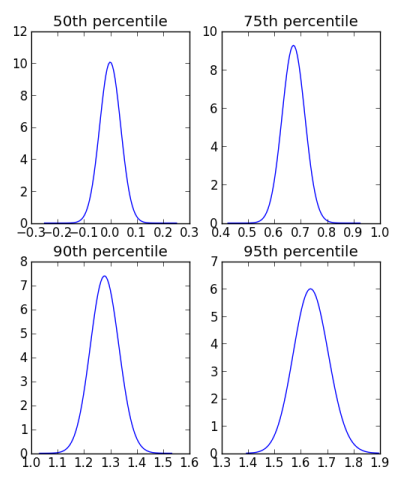# How variable are percentiles?

Suppose you’re trying to study the distribution of something by simulation. The average of your simulation values gives you an estimate of the mean value of the thing you’re simulating.

Next you want to have an idea how much the thing you’re simulating varies. You estimate the percentiles of your distribution by the percentiles of your samples. How variable are your estimates? For example, you estimate the 90th percentile of your distribution by finding the 90th percentile of your samples. How much is your estimate likely to change if you rerun you simulation with a new random number generator seed?

How does the variability of the percentiles depend on the percentile you’re looking at? For example, you might expect the median, i.e. the 50th percentile, to be the most stable. And as you start looking at more extreme percentiles, say the 5th or 95th, you might expect more variability. But how much more variability? A lot more or a little more?

To explore the questions above, let’s suppose we’re sampling from a standard normal distribution. (Of course if we really were sampling from something so well known as a normal distribution, we wouldn’t be doing simulation. But we need to pick something for illustration, so let’s use something simple.)

Suppose we simulate n values, sort them, and take the kth largest value.  This is called the kth order statistic. For example, we might estimate the 90th percentile of our distribution by using n = 1000 and k = 900. The distribution of the kth order statistic from n samples is known analytically (it’s a short derivation; see here) and so we can use it to make some plots.These plots use n = 1000. Each uses the same horizontal scale, so you can see how the distributions get wider as we move to higher percentiles. The distribution for the 95th percentile is about twice as wide as the distribution for the median.

Each plot is centered at the corresponding quantile for the standard normal distribution. For example, the plot for the distribution of the 75th percentile of the samples is centered at the 75th percentile of the standard normal.

The distribution for the sample median is unbiased, symmetric about 0. The distributions for the 75th and 90th percentiles are slightly biased to the left, i.e. they slightly underestimate the true values on average. The 95th percentile, however, is slightly biased in the opposite direction. [Update: As pointed out in the comments below, it seems the appearance of bias was a plotting artifact.]

If you’d like to see the details of how the plot was made, here is the Python code.

```import scipy as sp
import scipy.stats
import scipy.special
import matplotlib.pyplot as plt

def pdf(y, n, k):
Phi = scipy.stats.norm.cdf(y)
phi = scipy.stats.norm.pdf(y)
return scipy.stats.beta.pdf(Phi, k, n+1-k)*phi

def plot_percentile(p):
center = scipy.stats.norm.ppf(0.01*p)
t = sp.linspace(center-0.25, center+0.25, 100)
plt.plot(t, pdf(t, 1000, 10*p))
plt.title( "{0}th percentile".format(p) )

plt.subplot(221)
plot_percentile(50)
plt.subplot(222)
plot_percentile(75)
plt.subplot(223)
plot_percentile(90)
plt.subplot(224)
plot_percentile(95)
plt.show()
```## 6 thoughts on “How variable are percentiles?”

1. I’ll comment. If we simulate a mathematical blob like the 3x+1 function, then statistical models are really just non-rigorous guessing games that we honestly cannot “expect” to mean anything at all.

When simulating something real world, it seems like an error budget is a key part of any model. For example, a great error buget shows us that the pioneer spaceceaft saw what we can call an anomalous acceleration towards the sun. A closer look a TWT operation tells us that no matter how well the tube design, there exists a parasitic effect, see Pierce, causing f_out to always be greater than f_in. This effect looks big enough to explain the anomaly, i.e., would look like a blue shift, would show up in the signal similar to the doppler effect, and further there was a change in the magnatude of the effect when one craft switched to the back-up tube.

2. the cyclist

Apologies if I am mistaken here, but …

The 50th, 75th, 90th, and 95th percentiles of the standard normal are approximately 0; 0.6745; 1.2816; and 1.6449, respectively.

To my eye, it looks to me that your plots are NOT actually centered on those values, and the distributions ARE centered on those values (and perhaps not biased after all).

I am not a Python expert, but it looks like you were trying to plot an interval of width 0.25 units on each side of the center, for a total width of 0.5; but your plots are all 0.6 units wide, so maybe Python did something behind your back?

Sorry if I am totally off-base here.

3. Andrew Au

I have difficulty understanding the paper, what is the captial Phi function in equation (2)?

4. Andrew: Phi is the cumulative density function of a standard normal random variable. And phi (lower case) is its derivative, the corresponding probability density function.

You can read more detail here.

5. John, those plots are a bit confusing. The x scale is the same but the values vary and the centring of the plots is not quite the same. More importantly, though, the y scales vary too, so I can’t really compare their heights. If the heights aren’t really there to be compared, normalize them to the same height.

Ideally though, wouldn’t these be on one plot? Normalized, centred, etc. Then I can see the how much wider they get.

Perhaps all that’s needed is a simple plot of the FWHM value for all these distributions versus the percentage?

6. Lucia

What happend with the binomial? Make sense to do the percentil histograms?